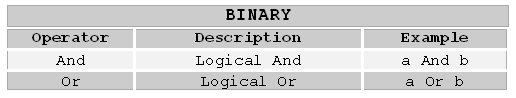## 9.4 Logical operators

Hollywood supports the following logical operators:The binary logical operators allow you to make decisions based on multiple conditions. Each binary logical operator needs two operands which are used to evaluate the result of the logical condition. All values that are not 0, Nil or the empty string ("") are considered True.

The `And` and `Or` operators use short-cut evaluation. This means that if the first operand already defines the result, the second operand is not evaluated at all. For example, if the first operand of an `And` expression is False (zero), then the second operand does not need to be evaluated because the whole expression cannot be True anyway. The same applies to an `Or` expression if the first operand of it is True (non-zero). Then the whole expression will always be True - no matter what value the second operand has.

Please note: `And` and `Or` do not return the constant True (1) if they are true. `And` returns the second operand if it is true and `Or` returns the first operand if it is true. For example:

 ```a = 5 And 4 ; a = 4 a = 5 And 0 ; a = 0 a = 0 And 4 ; a = 0 b = 5 Or 4 ; b = 5 b = 5 Or 0 ; b = 5 b = 0 Or 4 ; b = 4 ```

The unary `Not` operator will negate its operand. The result will always be True (1) or False (0). If used on a string it will result in True if the string is empty (""). For example:

 ```a = Not True ; a = 0 (False) a = Not False ; a = 1 (True) a = Not 5 ; a = 0 (False) a = Not Not True ; a = 1 (True) a = Not "Hello" ; a = 0 (False) a = Not "" ; a = 1 (True) ```

Please note: The `Not` operator has a high priority. You will need parentheses in most cases. For example, this does not work:

 ```If Not a = -1 ; wrong! ```

Hollywood will translate it to

 ```If (Not a) = -1 ```

because the `Not` operator has a higher priority than the equality operator! But obviously this translation does not make any sense because the result of the expression in parentheses (Not a) will always be 0 or 1 but never ever -1. Therefore, if you would like to check if a is not -1, you will have to use parentheses around the expression with the lower priority:

 ```If Not (a = -1) ; correct! ```

See Operator priorities for details.

Show TOC